Skip to content
Related Articles
Maximum Subarray Sum in a given Range
• Difficulty Level : Hard
• Last Updated : 09 May, 2020

Given an array of n numbers, the task is to answer the following queries:

```maximumSubarraySum(start, end) : Find the maximum
subarray sum in the range from array index 'start'
to 'end'.
```

Also see : Range Query With Update Required

Examples:

```Input : arr[] = {1, 3, -4, 5, -2}
Query 1: start = 0, end = 4
Query 2: start = 0, end = 2
Output : 5
4
Explanation:
For Query 1, [1, 3, -4, 5] or (  )
represent the maximum sum sub arrays
with sum = 5.

For Query 2, [1, 3] represents the
maximum sum subarray in the query range
with sum = 4
```

Segment Trees can be used to solve this problem.Here, we need to keep information regarding various cumulative sums.At every Node we store the following:
1) Maximum Prefix Sum,
2) Maximum Suffix Sum,
3) Total Sum,
4) Maximum Subarray Sum

A classical Segment Tree with each Node storing the above information should be enough to aswer each query. The only focus here is on how the left and the right Nodes of the tree are merged together. Now, we will discuss how each of the information is constructed in each of the segment tree Nodes using the information of its left and right child.

Constructing the Maximum Prefix Sum using Left and Right child

There can be two cases for maximum prefix sum of a Node:

1. The maximum prefix sum occurs in the left child,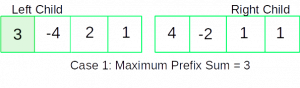```In this Case,
Maximum Prefix Sum = Maximum Prefix Sum of Left Child
```
2. The maximum prefix sum contains every array element of the left child and the elements contributing to the maximum prefix sum of the right child,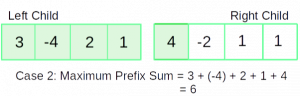```In this Case,
Maximum Prefix Sum = Total Sum of Left Child +
Maximum Prefix Sum of Right Child
```

Constructing the Maximum Suffix Sum using Left and Right child

There can be two cases for maximum suffix sum of a Node:

1. The maximum suffix sum occurs in the right child,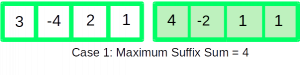```In this Case,
Maximum Suffix Sum = Maximum Suffix Sum of Right Child
```
2. The maximum suffix sum contains every array element of the Right child and the elements contributing to the maximum suffix sum of the left child,```In this Case,
Maximum Suffix Sum = Total Sum of Right Child +
Maximum Suffix Sum of Left Child
```

Constructing the Maximum Subarray Sum using Left and Right child

There can be three cases for the maximum sub-array sum of a Node:

1. The maximum sub-array sum occurs in the left child,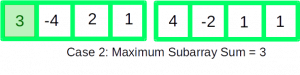```In this Case,
Maximum Sub-array Sum = Maximum Subarray Sum of Left Child
```
2. The maximum sub-array sum occurs in the right child,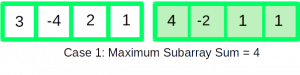```In this Case,
Maximum Sub-array Sum = Maximum Subarray Sum of Right Child
```
3. The maximum subarray sum, contains array elements of the right child contributing to the maximum prefix sum of the right child, and the array elements of the Left child contributing to the maximum suffix sum of the left child,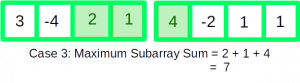```In this Case,
Maximum Subarray Sum = Maximum Prefix Sum of Right Child
+
Maximum Suffix Sum of Left Child
```
 `// C++ Program to Implement Maximum Sub-Array Sum in a range``#include ``using` `namespace` `std;`` ` `#define inf 0x3f3f`` ` `/* Node of the segment tree consisting of:``1. Maximum Prefix Sum,``2. Maximum Suffix Sum,``3. Total Sum,``4. Maximum Sub-Array Sum */``struct` `Node {``    ``int` `maxPrefixSum;``    ``int` `maxSuffixSum;``    ``int` `totalSum;``    ``int` `maxSubarraySum;``  ` `    ``Node()``    ``{``        ``maxPrefixSum = maxSuffixSum = maxSubarraySum = -inf;``        ``totalSum = -inf;``    ``}``};``  ` `// Returns Parent Node after merging its left and right child``Node merge(Node leftChild, Node rightChild)``{``    ``Node parentNode;``    ``parentNode.maxPrefixSum = max(leftChild.maxPrefixSum,``                                  ``leftChild.totalSum + ``                                  ``rightChild.maxPrefixSum);``  ` `    ``parentNode.maxSuffixSum = max(rightChild.maxSuffixSum,``                                  ``rightChild.totalSum +``                                  ``leftChild.maxSuffixSum);``  ` `    ``parentNode.totalSum = leftChild.totalSum +``                          ``rightChild.totalSum;``  ` `    ``parentNode.maxSubarraySum = max({leftChild.maxSubarraySum,``                                     ``rightChild.maxSubarraySum,``                                     ``leftChild.maxSuffixSum + ``                                     ``rightChild.maxPrefixSum});``  ` `    ``return` `parentNode;``}``  ` `// Builds the Segment tree recursively``void` `constructTreeUtil(Node* tree, ``int` `arr[], ``int` `start,``                                    ``int` `end, ``int` `index)``{``  ` `    ``/* Leaf Node */``    ``if` `(start == end) {``  ` `        ``// single element is covered under this range``        ``tree[index].totalSum = arr[start];``        ``tree[index].maxSuffixSum = arr[start];``        ``tree[index].maxPrefixSum = arr[start];``        ``tree[index].maxSubarraySum = arr[start];``        ``return``;``    ``}``  ` `    ``// Recursively Build left and right children``    ``int` `mid = (start + end) / 2;``    ``constructTreeUtil(tree, arr, start, mid, 2 * index);``    ``constructTreeUtil(tree, arr, mid + 1, end, 2 * index + 1);``  ` `    ``// Merge left and right child into the Parent Node``    ``tree[index] = merge(tree[2 * index], tree[2 * index + 1]);``}``  ` `/* Function to construct segment tree from given array. ``   ``This function allocates memory for segment tree and ``   ``calls constructTreeUtil() to fill the allocated ``   ``memory */``Node* constructTree(``int` `arr[], ``int` `n)``{``    ``// Allocate memory for segment tree``    ``int` `x = (``int``)(``ceil``(log2(n))); ``// Height of the tree``  ` `    ``// Maximum size of segment tree ``    ``int` `max_size = 2 * (``int``)``pow``(2, x) - 1; ``    ``Node* tree = ``new` `Node[max_size];``  ` `    ``// Fill the allocated memory tree``    ``constructTreeUtil(tree, arr, 0, n - 1, 1);``  ` `    ``// Return the constructed segment tree``    ``return` `tree;``}``  ` `/* A Recursive function to get the desired ``   ``Maximum Sum Sub-Array,``The following are parameters of the function-``  ` `tree     --> Pointer to segment tree ``index --> Index of the segment tree Node ``ss & se  --> Starting and ending indexes of the ``             ``segment represented by``                 ``current Node, i.e., tree[index]``qs & qe  --> Starting and ending indexes of query range */``Node queryUtil(Node* tree, ``int` `ss, ``int` `se, ``int` `qs,``                               ``int` `qe, ``int` `index)``{``    ``// No overlap``    ``if` `(ss > qe || se < qs) {``  ` `        ``// returns a Node for out of bounds condition``        ``Node nullNode;``        ``return` `nullNode;``    ``}``  ` `    ``// Complete overlap``    ``if` `(ss >= qs && se <= qe) {``        ``return` `tree[index];``    ``}``  ` `    ``// Partial Overlap Merge results of Left ``    ``// and Right subtrees``    ``int` `mid = (ss + se) / 2;``    ``Node left = queryUtil(tree, ss, mid, qs, qe, ``                                     ``2 * index);``    ``Node right = queryUtil(tree, mid + 1, se, qs, ``                              ``qe, 2 * index + 1);``  ` `    ``// merge left and right subtree query results``    ``Node res = merge(left, right);``    ``return` `res;``}``  ` `/* Returns the Maximum Subarray Sum between start and end``   ``It mainly uses queryUtil(). */``int` `query(Node* tree, ``int` `start, ``int` `end, ``int` `n)``{``    ``Node res = queryUtil(tree, 0, n - 1, start, end, 1);``    ``return` `res.maxSubarraySum;``}``  ` `int` `main()``{``    ``int` `arr[] = { 1, 3, -4, 5, -2 };``    ``int` `n = ``sizeof``(arr) / ``sizeof``(arr);``  ` `    ``// Construct Segment Tree``    ``Node* Tree = constructTree(arr, n);``    ``int` `start, end, maxSubarraySum;``  ` `    ``// Answering query 1:``    ``start = 0;``    ``end = 4;``    ``maxSubarraySum = query(Tree, start, end, n);``    ``cout << ``"Maximum Sub-Array Sum between "``         ``<< start << ``" and "` `<< end``         ``<< ``" = "` `<< maxSubarraySum << ``"\n"``;``  ` `    ``// Answering query 2:``    ``start = 0;``    ``end = 2;``    ``maxSubarraySum = query(Tree, start, end, n);``    ``cout << ``"Maximum Sub-Array Sum between "``         ``<< start << ``" and "` `<< end``         ``<< ``" = "` `<< maxSubarraySum << ``"\n"``;``  ` `    ``return` `0;``}`
Output:
```Maximum Sub-Array Sum between 0 and 4 = 5
Maximum Sub-Array Sum between 0 and 2 = 4
```

Time Complexity: O(logn) for each query.

Attention reader! Don’t stop learning now. Get hold of all the important DSA concepts with the DSA Self Paced Course at a student-friendly price and become industry ready.  To complete your preparation from learning a language to DS Algo and many more,  please refer Complete Interview Preparation Course.

In case you wish to attend live classes with industry experts, please refer DSA Live Classes

My Personal Notes arrow_drop_up